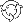The Theory \ Details \ portfolio analytics \ analytical portfolio

# Portfolio Analytics

## Analytical portfolio

### Dynamics of Portfolio Wealth, generated by Portfolio Strategy with Constant Weights

Assume that discounted prices of assets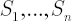move according to the analytical model with Mu vector, vector of continuously compounded dividend yields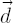, covariance matrixand risk-free rate. For
simplicity assume that the vector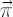is constant through time. Then dynamics of discounted portfolio wealth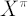, corresponding to portfolio vector, satisfies the
following equation: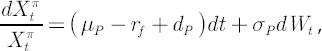where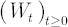is a
Wiener process,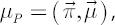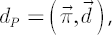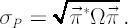Note.
Readers who are not familiar with stochastic differential equations in continuous time may interpret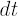as very short time range,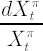as a
simple return on portfolio overand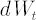as a normally
distributed random variable with zero mean and variance, independent of other variables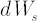,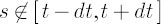.

Definition. Quantity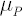is called Portfolio Mu, or Portfolio Expected Instantaneous Simple Rate of Return.
Definition. Quantity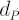is called Portfolio Dividend Yield.
Definition. Quantity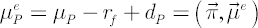is called Portfolio Excess Mu.
Definition. Quantityis called Portfolio Volatility.
Definition. Quantity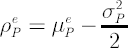is called
Portfolio Expected Excess Growth Rate.
The latter definition reflects the easily derived equality.

### Decomposition of Portfolio Variance

The direct consequence of the portfolio volatility definition is the following decomposition of portfolio variance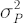: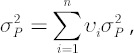where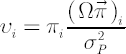. Obviously,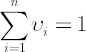Elementdenotes proportion of portfolio variance, contributed by-th asset.
In other words, vector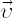with components, called Contributions to Portfolio Risk, determines alternative representation of portfolio structure, measured in units of portfolio variance rather than wealth, as in case of vector.